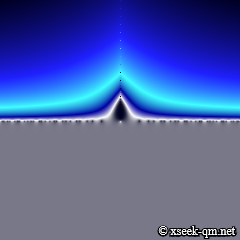# Mystery of the existence of the world

2014/10/4
Published 2010/12/3

"Everything is Number." ――― Pythagoras (c. 570 BC – c. 495 BC)Zeros of the zeta function

I thought the following things at a time in the past.

• The world is not a number.
• The physical world exists.
• We can modify the physical world by free will.

So I thought the following things for a long time.

• When did the world start?
• Where does the world exist?

After that, I have revised the idea. This world is a mathematical fact. For example, it is a mathematical fact, like followings.

• 5 regular polyhedra exist.
• 26 sporadic simple groups exist.
• Infinitely many zeros of the zeta function exist on the straight line.

The largest sporadic simple group is called "Monster group."

Though Riemann hypothesis is not proved, Hardy (1914) and Hardy & Littlewood (1921) showed there are infinitely many zeros on the line of Re(s) = 1/2. Therefore, it is a mathematical fact that infinitely many zeros exist on the straight line.

Physicist Steven Weinberg said the following in 1984.

The Universe is an enormous direct product of representations of symmetry groups. It's hard to say it any more strongly than that.

I guess that the universe is a direct product of representations of 26 sporadic simple groups.

The mathematical fact always exists. It does not start at a given time. It does not need time for a mathematical fact to exist.

The mathematical fact always exists. It does not start at a given time. It does not need time for a mathematical fact to exist.

The mathematical fact exists everywhere. It does not move from a given place. It does not need a place for a mathematical fact to exist.

Let's imagine the world without space-time and materials. What kind of objects can exist there? If something exists there, it seems to be a mathematical fact only.

We can not modify the inner space of the world from the outside of the world because the world is a mathematical fact. The mathematical fact includes the life form who recognizes that this world is a mathematical fact. How much self-reference it is!

Finally, I would like to finish this topic by quoting from the article written by physicist Freeman Dyson in 1983.

I have a sneaking hope, a hope unsupported by any facts or any evidence, that sometime in the twenty first century physicists will stumble upon the Monster group, built in some unsuspected way into the structure of the universe.

Related Articles: